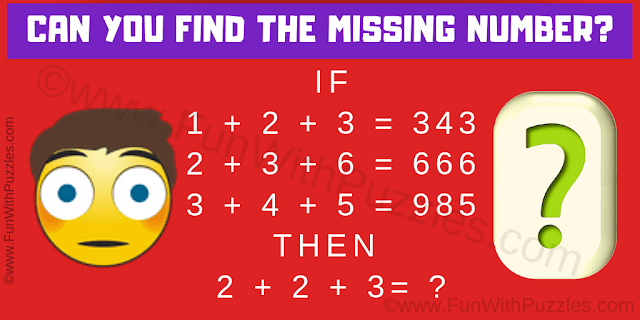This is a very interesting brain-twisting math logic question which will twist your brain. In this logic math question, there are a few number equations. Study these number equations carefully to decode the logical reasoning used in these equations. Apply the same logic to the last equation and find the value of the missing number which will replace the question mark.Can you find the missing number?
Answer of this "Brain Twisting Question", can be viewed by clicking on the answer button.